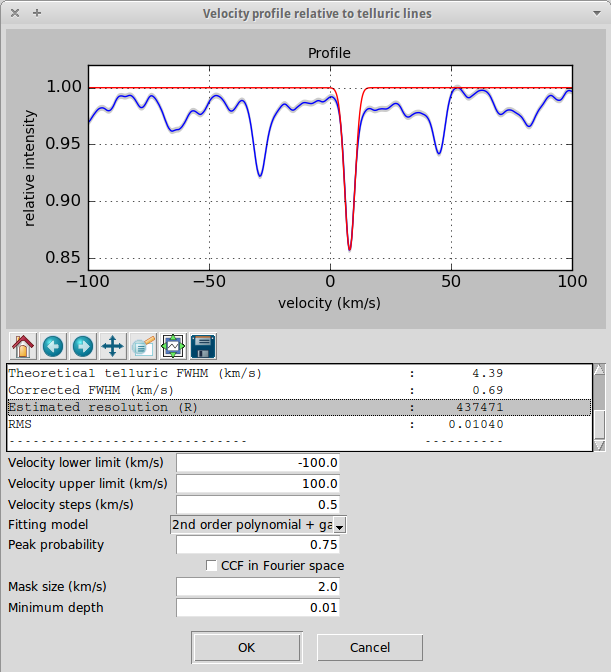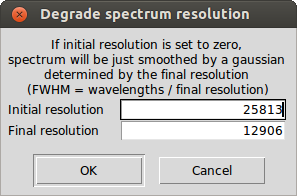# Resolution

## Resolution estimation

iSpec reports an estimate of the resolution based on the FWHM of the telluric lines.This estimation can be found in the velocity determination function relative to telluric lines and it is obtained by the following equation:

$R = \frac{c}{ (FWHM_{telluric} - FWHM_{theoretical}) }$

where $$c$$ is the speed of light in the vacuum (km/s), $$FWHM_{telluric}$$ (km/s) correspond to the telluric lines observed in the spectrum and $$FWHM_{theoretical}$$ (km/s) to the theoretical telluric lines.

The resolution is also reported when iSpec determines the velocity profile relative to the atomic data, however this is a worse estimator since it uses directly the FWHM measured and there are other effects (apart from the spectral resolution) that contribute to the broadening of the absorption lines (i.e. star's rotation).

In any case, it is preferable to know the real resolution of the spectra and not rely on these estimation since the error margin can be quite large.

The active spectrum resolution can be degraded to a lower one by selecting the menu "Operations - Degrade resolution" and selecting the original and target resolution. If the user has previously generated a velocity profile function, the current resolution has been already estimated and it will be shown in the degradation dialog (although the user can modify it).For each flux value, the process will:

• Define a window based on the FWHM size which depends on the original and target resolution
• Build a gaussian using the sigma value and the wavelength values of the spectra window
• Convolve the spectra window with the gaussian and save the convolved value

In case that the user specifies an initial resolution of zero, the spectrum will be just smoothed by a gaussian with variable FWHM determined by each wavelength and the final resolution:

$FWHM = \frac{wavelength}{ final\_resolution }$